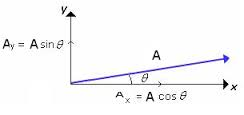# Pressure of an ideal gas

gracy
can someone help me .In this video from 2.00 to 3.00 how the velocity vector drawn with red color can be broken into x-y-z components and why after bouncing back only x component of velocity changed and y ,z components remained same?

Mentor
can someone help me .In this video from 2.00 to 3.00 how the velocity vector drawn with red color can be broken into x-y-z components and why after bouncing back only x component of velocity changed and y ,z components remained same?

It's bouncing off a y-z plane. Just bounce a ball off a vertical wall, and see which components reverse and which components remain the same. Only the component normal to the plane reverses.

Chet

•gracy
gracy
It's bouncing off a y-z plane. Just bounce a ball off a vertical wall, and see which components reverse and which components remain the same. Only the component normal to the plane reverses.

Chet
how the velocity vector drawn with red color can be broken into x-y-z components ?
It's bouncing off a y-z plane.
how you came to know that?

Mentor
how the velocity vector drawn with red color can be broken into x-y-z components ?
You can always resolve a vector into components.

how you came to know that?
You can see in the figure that the wall that it bounces off is parallel to the y-z plane.

Chet

gracy
You can always resolve a vector into components.
i know how to resolve vectors but only in 2 dimensions.

Mentor
i know how to resolve vectors but only in 2 dimensions.
Well, it can be done in 3 dimensions too.

Chet

gracy
Well, it can be done in 3 dimensions too.

Chet
can all vectors be resolved in 3 dimensions?

Mentor
can all vectors be resolved in 3 dimensions?
Yes. In 3D space, all vectors can be resolved into combinations of 3 linearly independent components.

Chet

gracy
Yes. In 3D space, all vectors can be resolved into combinations of 3 linearly independent components.

Chet
but only vectors which are in 3d ,for example this kind of vector (in the image below) can only be resolved in 2d i.e only with two perpendicular component.right?Mentor
but only vectors which are in 3d ,for example this kind of vector (in the image below) can only be resolved in 2d i.e only with two perpendicular component.right?All that means is that the component out of the paper is zero.

Chet

gracy
All that means is that the component out of the paper is zero.

Chet
ok if any vector is coming out of page ,what it's x component would be?i can only imagine it in y z plane.

Mentor
ok if any vector is coming out of page ,what it's x component would be?i can only imagine it in y z plane.
If the vector is through the origin, then you drop a normal from its tip to each of the three coordinate axes to get its x, y, and z components.

Chet

gracy
its tip
From Tip of vector which has to be resolved?

Mentor
From Tip of vector which has to be resolved?
Yes.